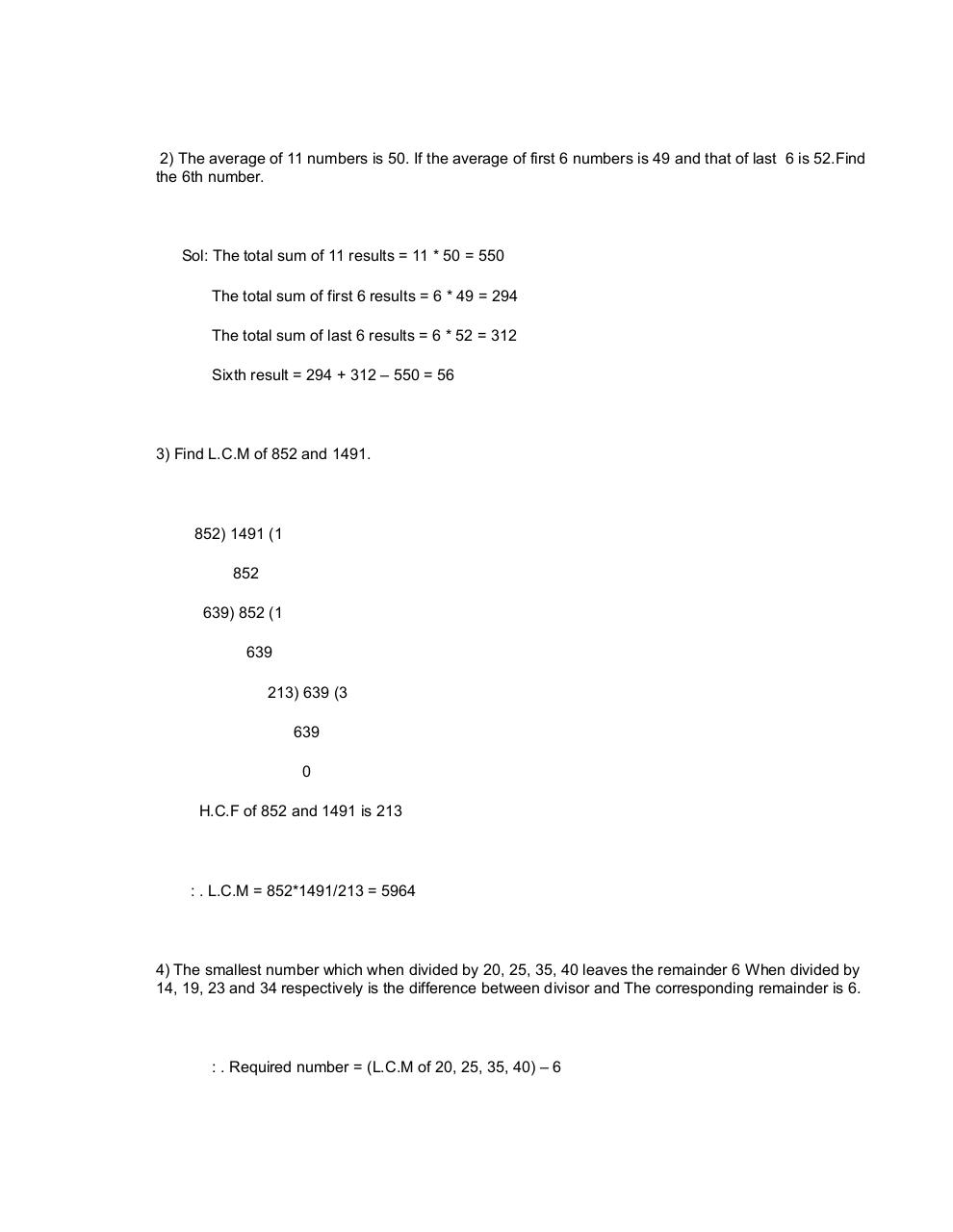# PDF Archive

Easily share your PDF documents with your contacts, on the Web and Social Networks.Page 1 2 3 45672

#### Text preview

2) The average of 11 numbers is 50. If the average of first 6 numbers is 49 and that of last 6 is 52.Find
the 6th number.

Sol: The total sum of 11 results = 11 * 50 = 550
The total sum of first 6 results = 6 * 49 = 294
The total sum of last 6 results = 6 * 52 = 312
Sixth result = 294 + 312 – 550 = 56

3) Find L.C.M of 852 and 1491.

852) 1491 (1
852
639) 852 (1
639
213) 639 (3
639
0
H.C.F of 852 and 1491 is 213

: . L.C.M = 852*1491/213 = 5964

4) The smallest number which when divided by 20, 25, 35, 40 leaves the remainder 6 When divided by
14, 19, 23 and 34 respectively is the difference between divisor and The corresponding remainder is 6.

: . Required number = (L.C.M of 20, 25, 35, 40) – 6